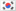532 1 2022年1月2日
5 1 投票

OA 通过之后就是要准备 VO 了，也就是所谓的面试。北美大厂的面试风格各不相同，所以下面我总结的面试技巧不见得能够应付所有的公司，但是以 Google 为代表的这种面试技巧，应付绝大多数公司的面试是毫无压力的。除了 Meta。

Meta 的话就只有一个方法：买 LeetCode 会员，一遍又一遍地刷他家公司 Tag 的题。

Technical 的 Interview 一般是 40 – 45 分钟，一旦开始，那就是要片刻不停地说，不要沉默太久。有些公司的面试官会有硬性指标，例如连续 15 秒或者 20 秒没有说话，就直接挂人了，但是大多数公司没有这么离谱的时间，主要看面试官的主观感受——你思考和沉默的时间是不是超过了预期值。

The next greater element of some element x in an array is the first greater element that is to the right of x in the same array.

You are given two distinct 0-indexed integer arrays nums1 and nums2, where nums1 is a subset of nums2.

For each 0 <= i < nums1.length, find the index j such that nums1[i] == nums2[j] and determine the next greater element of nums2[j] in nums2. If there is no next greater element, then the answer for this query is -1.

Return an array ans of length nums1.length such that ans[i] is the next greater element as described above.

Google 的题很少出现 Hard，基本以 Easy 和 Medium 为主。所以如何分析题目并展示解题思路就成了重中之重，而不仅仅是要写出一个能够运行的代码。

• We are asked to have a function, taking two parameters and return a positive integer.
• Given a string, the task asks me to have a solution to find the maximum number of substrings that satisfies …
• I will define a function to solve this problem. The input is an array with a positive integer indicating the array index, and the output is the sum of …

• Oh, so we are having an array of strings now, and they are guaranteed to be capitalized.
• And then we have a new definition, the next greater element.
• This sentence promises the answer must exist because …

• Since the problem says nums1 is a subset of nums2, we can know that all the integers of nums1 also appear in nums2, so if we iterate the elements in nums1, it is promised that we can find at least one elements in nums2 to be equal to that value.
• And by saying the “distinct”, does it mean all integers in nums1 and nums2 are unique? Is this understanding correct?

• What should I do if there is no valid answer? Do you want me to throw and exception? Or simply return a special value such as zero/negative one or negative infinity?
• Oh in this problem, this sentence tells us if we can’t find the next greater element, we should return a special value -1.

LeetCode 496 这道题原题给的 Constraints 是这样的：

• 1 <= nums1.length <= nums2.length <= 1000
• 0 <= nums1[i], nums2[i] <= 104
• All integers in nums1 and nums2 are unique.
• All the integers of nums1 also appear in nums2.

• With these clarifications, I will use BFS to solve this problem.
• This is a typical topological sorting problem, so I will use priority queue and XXX to solve it.
• Now I am going to use Union-Find to calculate the XXX.

• Here I want to use the example one to show my ideas. First I will iterate the array, [1, 3, 2], and for each element, at this point, it is 1, I doubled it, so it becomes 2, and …
• After we find the 2 in the stack we can pop it, and the stack is now [B, C] …
• 6 is less than 9 so we will stop the loop and return 6 as our final answer.

Follow Up 往往是加减一个条件，或者改一个条件，或者要求优化一类的，所以套路是一样的，理解题目的意思，然后指出之前的 Clarification 仍然成立的部分，并指出在修改或者增删条件之后，Clarification 不再成立的部分。例如：

• Without the constraint of XXX, we can’t …
• Since the elements is not unique now …
• The array is XXX so we can do it in a simpler way, but we need to care about two more edge cases.

“这个岗位需要有哪些知识储备？它平时是主要做什么工作？”

5 1 投票

1条留言（可选）如果您也有个人网站，不妨分享一下

1 评论ChuanboSafari 605.1.15 Mac OS X Lion 10_15_7

2022年1月7日 20:35